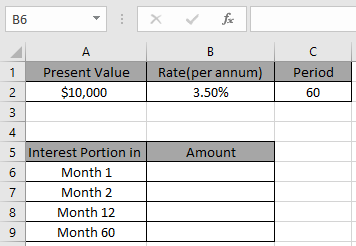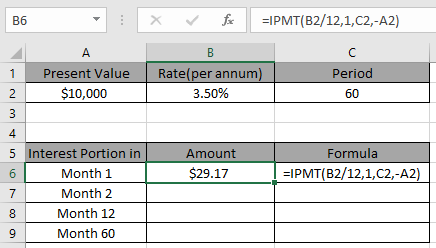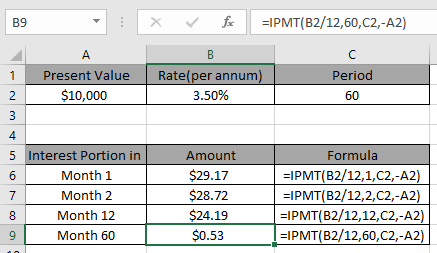# How to use the Excel IPMT Function

In this article, we will learn how to use the IPMT function in Excel.

You must be thinking when taking out a loan from a bank how much interest amount you owe to the bank. You can calculate the amount using Excel IPMT function.

Excel IPMT function returns the amount of interest portion for a specific period.
Syntax:

=IPMT (rate, per, nper, pv, [fv], [type])

rate: Interest rate per period
Per: payment period of interest.
nper: total no. of payment period.
pv: present value or the total loan amount.
fv [optional]: Extra cash balance if required. Default to 0.
type [optional]: When payments are due. Default is 0

Let’s understand this function using it an example.
Here we have a data set and to get IPMT amountWe need to find the interest amount for 4 different months.

Use the formula to get the interest amount for first month

=IPMT( B2/12 , 1 , C2 , -A2 )

Explanation:
B2/12: rate is divied by 12 as we are calculating interest for monthly periods.
1 : per is 1 as we are calculating for the first month. If you wish to find for any specific period. Mention the number for period you wish to find the interest amount
C2 : total period to pay the amountNow we will change the per value to find the interest amount for a specific period.Hope you understood how to use the IPMT function in Excel. Explore more articles on Excel satistical function here. Please feel free to state your query or feedback for the above article.

Related Articles:

Simple interest formula in Excel

How to Use Compound Interest Function in Excel

Popular Articles:

How to use the VLOOKUP Function in Excel

How to use the COUNTIF function in Excel 2016

How to Use SUMIF Function in Excel

Terms and Conditions of use

The applications/code on this site are distributed as is and without warranties or liability. In no event shall the owner of the copyrights, or the authors of the applications/code be liable for any loss of profit, any problems or any damage resulting from the use or evaluation of the applications/code.